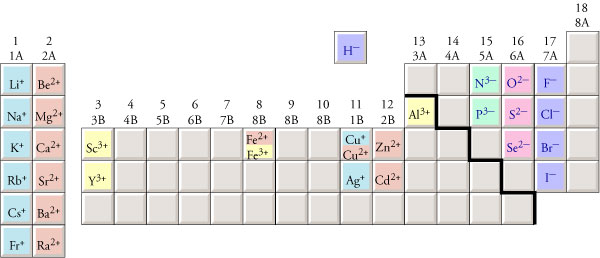﻿ Ionic Nomenclature Help

#Ionic Nomenclature Help

 Converting Formulas to Names Chemists have established different sets of rules for writing the names and formulas of different types of chemical compounds, so the first step in writing a name from a chemical formula is to decide what type of compound the formula represents. A chemical formula for an ionic compound will have one of the following forms. Metal-nonmetal: Ionic compounds whose formula contains one symbol for a metal and one symbol for a nonmetal are called binary ionic compounds. Their general formula is MaAb, with "M" representing the symbol of a metallic element, "A" representing the symbol for a nonmetallic element, and lowercase "a" and "b" representing subscripts in the formula (unless one or more of the subscripts are assumed to be 1). For example, NaF (used to fluoridate municipal waters), MgCl2 (used in floor sweeping compounds), and Al2O3 (in ceramic glazes) represent binary ionic compounds.Metal-polyatomic ion: Polyatomic ions can take the place of monatomic anions, so formulas that contain a symbol for a metallic element and the formula for a polyatomic ion represent ionic compounds. For example, NaNO3 (in solid rocket propellants) and Al2(SO4)3 (a foaming agent in fire foams) represent ionic compounds. Ammonium-nonmetal or ammonium-polyatomic ion: Ammonium ions, NH4+, can take the place of metallic cations in an ionic compound, so chemical formulas that contain the formula for ammonium with either a symbol for a nonmetallic element or a formula for a polyatomic ion represent ionic compounds. For example, NH4Cl (in dry cell batteries), (NH4)2S (used to color brass), and (NH4)2SO4 (in fertilizers) represent ionic compounds. The names of ionic compounds consist of the name for the cation followed by the name for the anion. Cations are named in one of the following ways. name of metal...for the metallic elements in Groups 1, 2, and 3 and for Al3+, Zn2+, Cd2+, and Ag+. name of metal(Roman numeral)...for the other metallic elements. name of polyatomic ion...the ammonium ion, NH4+, is the only common polyatomic cation. Anions are named in one of the following ways. (root of the name of a nonmetal)ide...for monatomic anions. name of polyatomic ion   See the following links to get more information on naming cations and anions. Converting Names to Formulas Before you can write a chemical formula from the name of a compound, you need to recognize what type of compound the name represents. For binary ionic compounds, the first part of the name is the name of a metallic cation. This may include a Roman numeral in parentheses. The anion name starts with the root of the name of a nonmetal and ends with ‑ide. (name of metal)(maybe Roman numeral) (root of nonmetal)ide For example, aluminum fluoride (used in the production of aluminum) and tin(II) chloride (used in galvanizing tin) are binary ionic compounds. You can identify other names as representing ionic compounds by recognizing that they contain the names of common polyatomic ions. For example, ammonium chloride and iron(III) hydroxide are both ionic compounds. Many of the polyatomic ions that you will be expected to recognize end in ‑ate, so this ending tells you that the name represents an ionic compound. Copper(II) sulfate is an ionic compound. Follow these steps to write formulas for ionic compounds. Step 1: Write the formula, including the charge, for the cation. Step 2: Write the formula, including the charge, for the anion. Step 3: Write subscripts for each formula so as to yield an uncharged compound. ( Use the lowest whole number ratio for the subscripts. If the subscript for a polyatomic ion is higher than one, place the formula for the polyatomic ion in parentheses and put the subscript outside the parentheses.) The image below shows the formulas for monatomic ions for which you should know the charges.You should know the names and formulas for the following polyatomic ions. hydroxide ion, OH- nitrate ion, NO3- acetate ion, C2H3O2- carbonate ion, CO32- sulfate ion, SO42- phosphate ion, PO43- Some polyatomic anions are formed by the attachment of one or more hydrogen atoms. In fact, it is common for hydrogen atoms to be transferred from one ion or molecule to another ion or molecule. When this happens, the hydrogen atom is usually transferred without its electron, as H+. If an anion has a charge of -2 or -3, it can gain one or two H+ ions and still retain a negative charge. For example, carbonate, CO32-, can gain an H+ ion to form HCO3-, which is found in baking soda. The sulfide ion, S2-, can gain one H+ ion to form HS-. Phosphate, PO43-, can gain one H+ ion and form HPO42-, or it can gain two H+ ions to form H2PO4-. These polyatomic ions are named with the word hydrogen in front of the name of the anion if there is one H+ ion attached and dihydrogen in front of the name of the anion if two H+ ions are attached. HCO3- is hydrogen carbonate ion. HS- is hydrogen sulfide ion. HPO42- is hydrogen phosphate ion. H2PO4- is dihydrogen phosphate ion. See the following links to get more information on writing formulas for\cations and anions.
Chiral Publishing Company